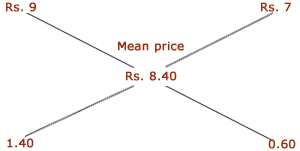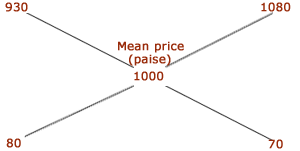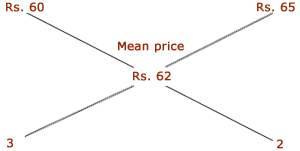## Arithmatical – Alligation or Mixture

DIRECTIONS : Important Facts and Formulae
15. How many kilograms of sugar costing Rs.9 per kg must be mixed with 27 kg of sugar costing Rs.7 per Kg so that there may be a gain of 10% by selling the mixture at Rs.9.24 per Kg? A.  36 Kg B.  42 Kg C.  54 Kg D.  63 Kg
Solution
 By the rule of alligation: C.P. of 1 kg sugar of 1st kind C.P. of 1 kg sugar of 2nd kindRatio of quantities of 1st and 2nd kind = 14 : 6 = 7 : 3. Let x kg of sugar of 1st kind be mixed with 27 kg of 2nd kind. Then, 7 : 3 = x : 27 or x = (7 x 27 / 3) = 63 kg.
16. In what ratio must rice at Rs.9.30 per Kg be mixed with rice at Rs.10.80 per Kg so that the mixture be worth Rs.10 per Kg? A.  8 : 7 B.  7 : 8 C.  8 : 9 D.  9 : 8
Solution
 By the rule of alligation: C.P. of 1 kg rice of 1st kind (in paise) C.P. of 1 kg rice of 2nd kind (in paise)Required ratio = 80 : 70 = 8 : 7.

17. In what ratio must be a grocer mix two varities of tea worth Rs.60 a kg and Rs.65 a Kg so that by selling the mixture at Rs.68.20 a Kg he may gain 10%? A.  3 : 4 B.  3 : 2 C.  3 : 5 D.  4 : 5
Solution
 S.P. of 1 kg of the mixture = Rs. 68.20, Gain = 10 % C.P. of 1 kg of the mixture = Rs. (100 / 110 x 68.20) = Rs. 62. By the rule of alligation: C.P. of 1 kg tea of 1st kind C.P. of 1 kg tea of 2nd kindRequired ratio = 3 : 2.
Page 4 of 4 123 4